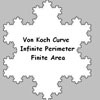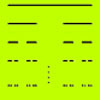#### You may also like### Sierpinski Triangle

What is the total area of the triangles remaining in the nth stage of constructing a Sierpinski Triangle? Work out the dimension of this fractal.### Von Koch Curve

Make a poster using equilateral triangles with sides 27, 9, 3 and 1 units assembled as stage 3 of the Von Koch fractal. Investigate areas & lengths when you repeat a process infinitely often.### How Many Elements Are There in the Cantor Set?

This article gives a proof of the uncountability of the Cantor set.

# Squareflake

##### Age 16 to 18Challenge Level

Imagine breaking a cube into 64 identical small cubes. The length of the edge of the big cube is 4 times the length of the edge of a small cube and we say that the magnification factor is 4. As $64=4^3$ we see that the number of small self similar pieces is equal to the magnification factor cubed. The number 3 is called the dimension of the cube.

If you break a line of length 1 into self similar bits of length ${1\over m}$ there are $m^1$ bits and the dimension of the line is 1.

If you break up a square of side 1 into self similar squares with edge ${1\over m}$ then there are $m^2$ smaller squares and the dimension is 2.

If you break up a cube of side 1 into self similar cubes with edge ${1\over m}$ then there are $m^3$ smaller cubes and the dimension is 3.

In each case we say the magnification factor is $m$ meaning that we have to scale the lengths by a factor of $m$ to produce the original shape. The formula for dimension is: $n = m^d$ where $n$ is the number of self similar bits, and $d$ is the dimension.

We can generalise what we know about 1, 2 and 3 dimensions to the non integer dimensions of fractals using the formula (where d is the dimension): $$\rm{number of self similar bits} = \rm{(magnification factor})^d.$$

You can download a free copy of MSW Logo fromhttp://www.softronix.com/logo.html

This is the program that draws the squareflake.

to flake :side :stage
repeat 4[line :side :stage rt 90]
end

to line :side :stage
if :stage = 0 [fd :side stop]
line :side /4 :stage - 1 lt 90
line :side /4 :stage - 1 rt 90
line :side /4 :stage - 1 rt 90
repeat 2 [line :side /4 :stage - 1] lt 90
line :side /4 :stage - 1 lt 90
line :side /4 :stage - 1 rt 90
line :side /4 :stage - 1
end